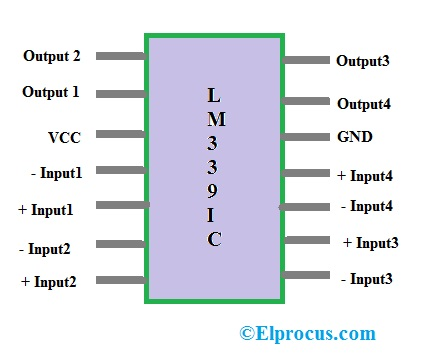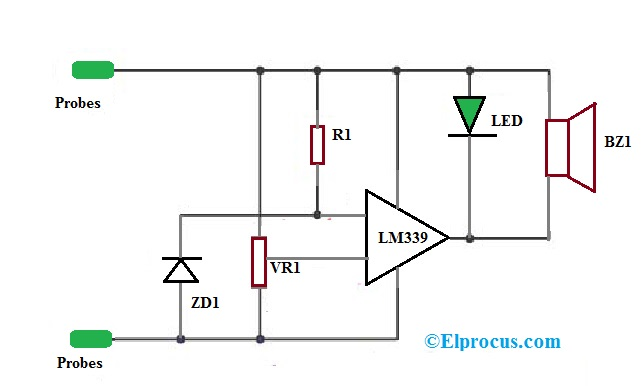# IC LM339 Pin Configuration, Circuit Diagram and Its Applications

The voltage comparator is one kind of integrated circuit, especially used for contrast two voltages or currents at the comparator two inputs. The main function of this IC is, the comparator has two inputs where it compares the two inputs with each other then generates a differential output like high-level signals or low-level signal. In general, comparators are classified into different types which include electronic, electrical, optical, sigma, mechanical, digital, pneumatic and many more comparators. The comparator circuit can be designed by using various basic electrical and electronic components like resistors, operational amplifiers, transistors, diodes, etc. All these comparators are applicable to making electrical and electronic projects.

## IC LM339 Pin Configuration

LM 339 IC has four inbuilt comparators. It is a 14-pin chip as shown in below pin configuration. This IC comprises four voltage comparators that are intended to work with only power supply. And also there will be a possibility of operation using a dual power supply, as long as the variation among the two voltages is 2 volts to 36 volts.LM339 IC pin Configuration
• Pin1 (OUT): It is an output pin of the first comparator.
• Pin2 (OUT): It is an output pin of the second comparator.
• Pin3 (VCC): It is a Power supply of the comparator.
• Pin4 (IN-): It is a negative input pin of the second comparator.
• Pin5 (IN+): It is a positive input pin of the second comparator.
• Pin6: (IN-): It is a negative input of the first comparator.
• Pin7: (IN+): It is a positive pin of the first comparator.
• Pin8: (IN-): It is a negative pin of the third comparator.
• Pin9: (IN+): It is a positive e pin of the third comparator.
• Pin810: (IN-): It is a negative pin of the fourth comparator.
• Pin11: (IN+): It is a positive input pin of the fourth comparator.
• Pin12: (GND): It is a ground pin
• Pin13: (OUT): It is an output pin of the fourth comparator.
• Pin8: (OUT): It is an output pin of the third comparator.

### IC LM339 based Voltage Monitor of a Battery

The circuit of voltage monitor of a battery uses an LM399 comparator and the required components like resistors, potentiometer, and diode. This circuit can be built with R1 resistor-1K, VR1 potentiometer-5K, LM339 Voltage comparator IC, Zener diode ZD1-6V, LED, Piezo buzzer BZ1, etc.

When the probes are connected to the 9V battery, the circuit will activate. The IC LM399 has positive and negative pins which are pin-3 and pin12 as shown in the circuit. Simultaneously, the voltage supply from the battery will flow through the potentiometer VR1 to the non- inverting terminals (pin5) of the IC.LM339 IC Voltage Comparator Circuit

Then the resistor R1 in the circuit restricts the flow of current to limit towards the 6V Zener diode at inverting terminals of IC (pin4). Both the inverting and non-inverting ways voltage s of the IC will compare the two voltages in the IC1. As indicators, Buzzer and LEDs are used. The resistor R2 which is connected among the buzzer, as well as diode, will control the flow of current through them.

Here the comparison of two voltages in the circuit can be done by the IC, and the output is provided as V0, and the circuit is supplied by the single voltage supply VCC. The comparison between the two voltages can be done based on the following conditions.

If the primary voltage is greater than the second voltage (V1>V2) then the output voltage will be VCC.

If the primary voltage is less than the second voltage (V1<V2) then the output voltage will be GND or 0. Depending on the output in the circuit, we can decide whether the primary voltage is higher than the secondary voltage at the output voltage.

When the primary voltage is higher than the 6Volts, the output will be at a high voltage state. So LED will not blink as well as Buzzer cannot make the sound. Because each their terminals are connected to the output pin of the IC and positive supply.

When the primary voltage is lower than the 6 volts, then the LED will glow as well as the buzzer will make the sound. The voltage level and circuit sensitivity can be adjusted by potentiometer VR1.

#### Packages of IC LM339

The LM339IC has different packages and few of them are discussed below.

• For LM339DG, the package will be SOIC-14 (lead (pb) free)
• For LM339DR2G, the package will be SOIC-14 (lead (pb) free)
• For LM339DTBR2G, the package will be TSSOP-14 (lead (pb) free)
• For LM339NG, the package will be PDIP-14 (lead (pb) free)

#### Features of IC LM339

The major features of LM339 IC mainly include low-input bias current, low-current supply, low-output saturation voltage, low-input offset current, etc. Some of the features with SI (system international) units are discussed below.

• Wide single supply voltage values range from +3Volts to 36Volts.
• Low supply current is 1.1 mA
• Low input offset current is 5 nA
• The low input bias current is 25 nA
• Low output saturation voltage is 250 mW
• The low input offset voltage is 1mV
• Well-suited outputs TTL, MOS, CMOS

#### IC LM339 Ratings

The power, current and voltage ratings of IC LM339 and their standard values with SI units are discussed below.

• The (Vcc) supply voltage is 36Volts
• The (VIDR) input voltage differential range is 30Volts
• The (Isc) output short circuit to GND is continuous
• The (VICMR) input common mode voltage will range from -0.3Volts to Vcc
• The (TA) operating temperature is -25oc to 85oc
• The (PD) power dissipation is 1mW.
• The (TJ) junction temperature is 150oc

### IC LM339 Applications

The applications of IC LM339 mainly include Power supervision, Industrial, Oscillators, Peak detectors, Voltage comparators, Measuring instruments, Logic voltage translation, Automotive, Driving CMOS, Low-Frequency Op-amp, Transducer Amplifier, Zero Crossing Detector, Limit comparator, Crystal controlled oscillator, Negative reference comparator, Driving TTL, etc

Thus, this is all about LM339 IC pin configuration and its application. From the above information, finally, we can conclude that the voltage comparator is from the family of LMx39x series & it is designed by many manufacturing industries.  This IC can be inbuilt with four separate voltage comparators and operates by using a single power supply. And also operates with dual supply also, and the difference between the two supplies V1 & V2 is 2 Volts to 36Volts.